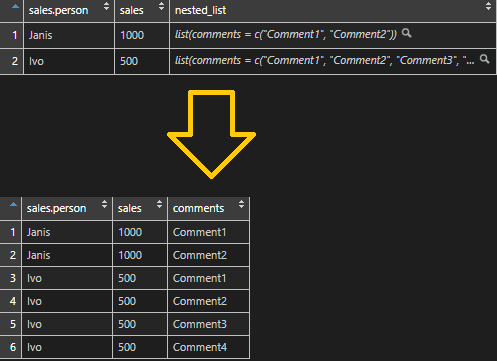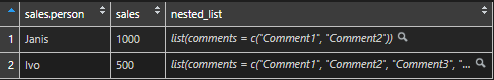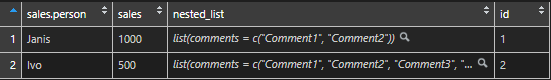# How to unlist a nested list in RHere is a simple tutorial on how to unlist a nested list with the help of R. Problems may appear when nested lists are a different length for each record. For example, chat sessions and corresponding lists of conversations that differ in length.

Here is all code altogether and additional explanations below.

```# create data frame with nested list
df <- data.frame("sales person" = c("Janis", "Ivo"),
"sales" = c(1000, 500))

nested_list <-  list(list("comments" = c("Comment1", "Comment2")),
list("comments" = c("Comment1", "Comment2", "Comment3", "Comment4"))
)

df_and_list <- mutate(df, nested_list )

require(data.table)
require(dplyr)

# unlist nested list with id
unlisted <- rbindlist(df_and_list\$nested_list, fill = T, idcol = "id")

# create same id in remaining data frame
df_and_list\$id <- seq.int(nrow(df_and_list))

# join data frame with unlisted list
df_and_list <- left_join(df_and_list, unlisted, by = "id")

#get rind of unnecessary columns
df_and_list\$nested_list <- NULL
df_and_list\$id <- NULL```

Create a simple data frame with a nested list.

```# create data frame with nested list
df <- data.frame("sales person" = c("Janis", "Ivo"),
"sales" = c(1000, 500))

nested_list <-  list(list("comments" = c("Comment1", "Comment2")),
list("comments" = c("Comment1", "Comment2", "Comment3", "Comment4"))
)

df_and_list <- mutate(df, nested_list )```With the help of package data.table function rbindlist create a data frame with an unlisted nested list column. Add id column, which is a key that shows the previous data frame row.

```require(data.table)
require(dplyr)

# unlist nested list with id
unlisted <- rbindlist(df_and_list\$nested_list, fill = T, idcol = "id")```Create a row id column in the first data frame.

```# create same id in remaining data frame
df_and_list\$id <- seq.int(nrow(df_and_list))```Join the first data frame and unlisted list data frame together.

```# join data frame with unlisted list
df_and_list <- left_join(df_and_list, unlisted, by = "id")```Here is another post dedicated to lists.

### 1 comment on “How to unlist a nested list in R”

1.Lisa Anders

Thank you for posting this! Really great.